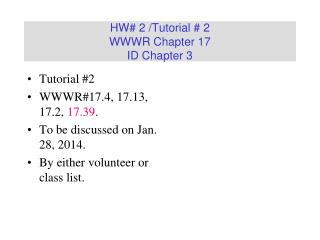# HW# 2 /Tutorial # 2 WWWR Chapter 17 ID Chapter 3 - PowerPoint PPT PresentationDownload PresentationHW# 2 /Tutorial # 2 WWWR Chapter 17 ID Chapter 3

HW# 2 /Tutorial # 2 WWWR Chapter 17 ID Chapter 3Download Presentation## HW# 2 /Tutorial # 2 WWWR Chapter 17 ID Chapter 3

- - - - - - - - - - - - - - - - - - - - - - - - - - - E N D - - - - - - - - - - - - - - - - - - - - - - - - - - -
##### Presentation Transcript

1. Tutorial #2 WWWR#17.4, 17.13, 17.2, 17.39. To be discussed on Jan. 28, 2014. By either volunteer or class list. HW# 2 /Tutorial # 2WWWR Chapter 17ID Chapter 3

2. Tutorial #2 WWWR #17.39: Line 2: The fins are made of aluminum, they are 0.3cm thick each. #17.2 The following correction should be made. (i) arithmetic mean area p(r0+ri) HW# 2 /Tutorial # 2Hints / Corrections

4. One-Dimensional Conduction Steady-state conduction, no internal generation of energy For one-dimensional, steady-state transfer by conduction i = 0 rectangular coordinates i = 1 cylindrical coordinates i = 2 spherical coordinates

5. 1/Rc=1/Ra+1/Rb Ra Rb Equivalent resistance of the parallel resistors Ra and Rb is Rc

6. Adapted from Heat and Mass Transfer – A Practical Approach, Y.A. Cengel, Third Edition, McGraw Hill 2007. Thus, insulating the pipe may actually increase the rate of heat transfer instead of decreasing it.

7. For steady-state conduction in the x direction without internal generation of energy, the equation which applies is Where k may be a function of T. In many cases the thermal conductivity may be a linear function temperature over a considerable range. The equation of such a straight-line function may be expressed by k = ko(1 + ßT) Where koand ß are constants for a particular material

8. . . q = qL [ 1 + ß (T - T L)] Plane Wall with Variable Energy Generation The symmetry of the temperature distribution requires a zero temperature gradient at x = 0. The case of steady-state conduction in the x direction in a stationary solid with constant thermal conductivity becomes

9. Detailed derivation for the transformation F = C + s q

10. Detailed Derivation for Equations 17-25 Courtesy by all CN5 Grace Mok, 2003-2004

11. Detailed Derivation for Equations 17-25 Courtesy by all CN5 Grace Mok, 2003-2004

12. Heat Transfer from Finned Surfaces • Temperature gradient dT/dx, • Surface temperature, T, • Are expressed such that T is a function of x only. • Newton’s law of cooling • Two ways to increase the rate of heat transfer: • increasing the heat transfer coefficient, • increase the surface area fins • Fins are the topic of this section. Adapted from Heat and Mass Transfer – A Practical Approach, Y.A. Cengel, Third Edition, McGraw Hill 2007.

13. Heat transfer from extended surfaces

14. (A) For constant cross section and constant thermal conductivity Where • Equation (A) is a linear, homogeneous, second-order differential equation with constant coefficients. • The general solution of Eq. (A) is • C1 and C2 are constants whose values are to be determined from the boundary conditions at the base and at the tip of the fin. (B)

15. Boundary Conditions Several boundary conditions are typically employed: • At the fin base • Specified temperatureboundary condition, expressed as: q(0)= qb=Tb-T∞ • At the fin tip • Specified temperature • Infinitely Long Fin • Adiabatic tip • Convection (and combined convection). Adapted from Heat and Mass Transfer – A Practical Approach, Y.A. Cengel, Third Edition, McGraw Hill 2007.

16. How to derive the functional dependence of for a straight fin with variable cross section area Ac = A = A(x)?

17. General Solution for Straight Fin with Three Different Boundary Conditions

18. In set(a) Known temperature at x = L In set(b) Temperature gradient is zero at x = L In set(c) Heat flow to the end of an extended surface by conduction be equal to that leaving this position by convection.

19. Detailed Derivation for Equations 17-36 (Case a). Courtesy by CN3 Yeong Sai Hooi 2002-2003

20. Detailed Derivation for Equations 17-38 (Case b for extended surface heat transfer). Courtesy by CN3 Yeong Sai Hooi, 2002-2003

21. Detailed Derivation for Equations 17-40 (Case c for extended surface heat transfer). Courtesy by all CN4 students, presented by Loo Huiyun, 2002-2003

22. Detailed Derivation for Equations 17-46 (Case c for extended surface heat transfer). Courtesy by all CN4 students, presented by Loo Huiyun, 2002-2003

23. Infinitely Long Fin (Tfintip=T) Adapted from Heat and Mass Transfer – A Practical Approach, Y.A. Cengel, Third Edition, McGraw Hill 2007. • For a sufficiently long fin the temperature at the fin tip approaches the ambient temperature Boundary condition:q(L→∞)=T(L)-T∞=0 • When x→∞ so does emx→∞ C1=0 • @ x=0: emx=1 C2= qb • The temperature distribution: • heat transfer from the entire fin

24. Actual heat transfer rate from the fin Ideal heat transfer rate from the fin if the entire fin were at base temperature Fin Efficiency Adapted from Heat and Mass Transfer – A Practical Approach, Y.A. Cengel, Third Edition, McGraw Hill 2007. • To maximize the heat transfer from a fin the temperature of the fin should be uniform (maximized) at the base value of Tb • In reality, the temperature drops along the fin, and thus the heat transfer from the fin is less • To account for the effect we define a fin efficiency or